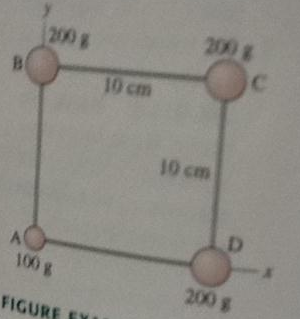# Problem: Four masses are connected by massless rigid rods (see figure).a. Find the coordinate of the center of mass.b. Find the moment of inertia about a diagonal axis that passes through B and D.

###### FREE Expert Solution

a.

Center of the mass of a system:

$\overline{){{\mathbf{r}}}_{\mathbf{c}\mathbf{m}}{\mathbf{=}}{\mathbf{\left(}}\frac{{\mathbf{m}}_{\mathbf{1}}{\mathbf{x}}_{\mathbf{1}}\mathbf{+}{\mathbf{m}}_{\mathbf{2}}{\mathbf{x}}_{\mathbf{2}}\mathbf{+}\mathbf{.}\mathbf{.}\mathbf{.}\mathbf{+}{\mathbf{m}}_{\mathbf{n}}{\mathbf{x}}_{\mathbf{n}}}{{\mathbf{m}}_{\mathbf{1}}\mathbf{+}{\mathbf{m}}_{\mathbf{2}}\mathbf{+}\mathbf{.}\mathbf{.}\mathbf{.}\mathbf{+}{\mathbf{m}}_{\mathbf{n}}}{\mathbf{,}}\frac{{\mathbf{m}}_{\mathbf{1}}{\mathbf{y}}_{\mathbf{1}}\mathbf{+}{\mathbf{m}}_{\mathbf{2}}{\mathbf{y}}_{\mathbf{2}}\mathbf{+}\mathbf{.}\mathbf{.}\mathbf{.}\mathbf{+}{\mathbf{m}}_{\mathbf{n}}{\mathbf{y}}_{\mathbf{n}}}{{\mathbf{m}}_{\mathbf{1}}\mathbf{+}{\mathbf{m}}_{\mathbf{2}}\mathbf{+}\mathbf{.}\mathbf{.}\mathbf{.}\mathbf{+}{\mathbf{m}}_{\mathbf{n}}}{\mathbf{\right)}}}$

80% (24 ratings)###### Problem Details

Four masses are connected by massless rigid rods (see figure).a. Find the coordinate of the center of mass.

b. Find the moment of inertia about a diagonal axis that passes through B and D.